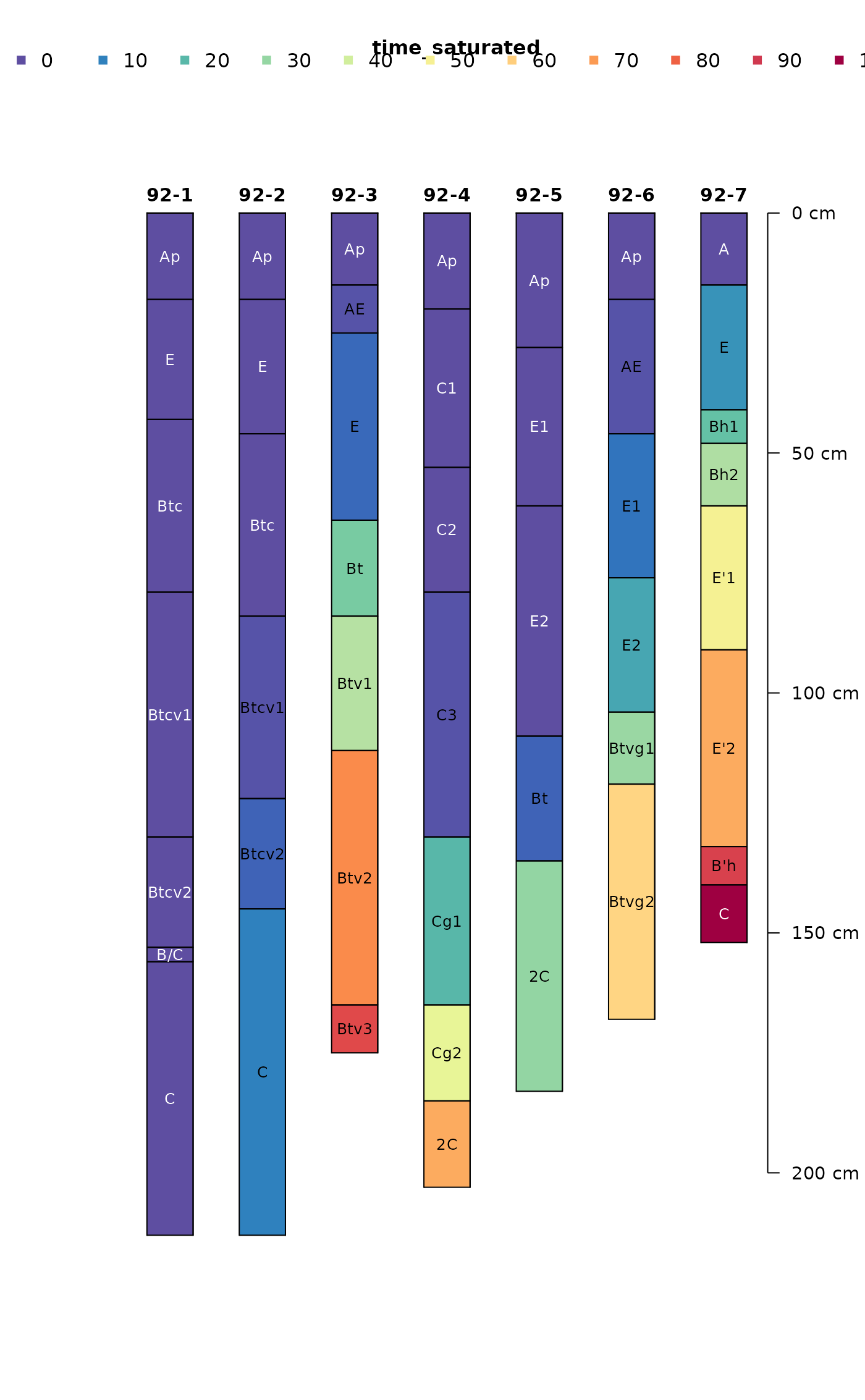Evaluate missing data in a SoilProfileCollection object

evalMissingData(
x,
vars,
name = hzdesgnname(x),
p = "Cr|R|Cd",
method = "relative"
)

## Arguments

x

a SoilProfileCollection object

vars

a character vector naming horizon-level attributes in x

name

the name of a horizon-level attribute where horizon designations are stored

p

REGEX pattern used to match non-soil horizons

method

'relative' (proportion of total) or 'absolute' depths

## Value

A vector values ranging from 0 to 1 (method='relative') or 0 to maximum depth in specified depth units (method='absolute'), representing the quantity of non-NA data (as specified in vars) for each profile.

## Details

Data completeness is evaluated by profile, based on the thickness of horizons with complete horizon-level attribute values (specified in vars) divided by the total thickness. The default REGEX pattern, p, should catch most non-soil horizons which are excluded from the evaluation.

D.E. Beaudette

## Examples


# example data
data(sp2)

# init SPC object
depths(sp2) <- id ~ top + bottom

# compute data completeness
sp2$data.complete <- evalMissingData(sp2, vars = c('r', 'g', 'b'), name = 'name') sp2$data.complete.abs <- evalMissingData(sp2, vars = c('r', 'g', 'b'),
name = 'name', method = 'absolute')

# rank
new.order <- order(sp2$data.complete) # plot along data completeness ranking plot(sp2, plot.order=new.order, name='name') # add relative completeness axis # note re-ordering of axis labels axis(side=1, at=1:length(sp2), labels = round(sp2$data.complete[new.order], 2),
line=-1.5, cex.axis=0.75)